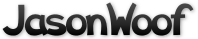Got questions, comments, patches, etc.? Contact Jason Woofenden
 author Joshua Grams Mon, 3 Jan 2005 01:49:56 +0000 (01:49 +0000) committer Joshua Grams Mon, 3 Jan 2005 01:49:56 +0000 (01:49 +0000)
xship/yship -> shipx/shipy
xvel/yvel -> shipdx/shipdy

 main.c patch | blob | history

diff --git a/main.c b/main.c
index ab97cbc..4801df7 100644 (file)
--- a/main.c
+++ b/main.c
@@ -45,7 +45,7 @@ struct rock_struct {
// Array of black pixel coordinates. This is scanned
// every frame to see if it's still black, and as
// soon as it isn't we BLOW UP
// Array of black pixel coordinates. This is scanned
// every frame to see if it's still black, and as
// soon as it isn't we BLOW UP
-       float x,y,xvel,yvel;
+       float x,y,dx,dy;
int active;
int dead;  // has been blown out of the way
// to make room for a new ship appearing.
int active;
int dead;  // has been blown out of the way
// to make room for a new ship appearing.
@@ -108,8 +108,8 @@ struct spacedot sdot[MAXSPACEDOTS];
char topline;
char *initerror = "";

char topline;
char *initerror = "";

-float xship,yship = 240.0;     // X position, 0..XSIZE
-float xvel,yvel;       // Change in X position per tick.
+float shipx,shipy = 240.0;     // X position, 0..XSIZE
+float shipdx,shipdy;   // Change in X position per tick.
float rockrate,rockspeed;
float movementrate;  // this controls the speed of everything that moves.
float yscroll;
float rockrate,rockspeed;
float movementrate;  // this controls the speed of everything that moves.
float yscroll;
@@ -193,7 +193,7 @@ init_space_dots() {
}

void
}

void
-makebangdots(int xbang, int ybang, int xvel, int yvel, SDL_Surface *s, int power) {
+makebangdots(int xbang, int ybang, int dx, int dy, SDL_Surface *s, int power) {

// TODO - stop generating dots after a certain amount of time has passed, to cope with slower CPUs.
// TODO - generate and display dots in a circular buffer

// TODO - stop generating dots after a certain amount of time has passed, to cope with slower CPUs.
// TODO - generate and display dots in a circular buffer
@@ -221,8 +221,8 @@ makebangdots(int xbang, int ybang, int xvel, int yvel, SDL_Surface *s, int power

r = 1-(rnd()*rnd());

r = 1-(rnd()*rnd());

-                                       bdot[bd2].dx = (power/50.0)*45.0*cos(theta)*r + xvel;
-                                       bdot[bd2].dy = (power/50.0)*45.0*sin(theta)*r + yvel;
+                                       bdot[bd2].dx = (power/50.0)*45.0*cos(theta)*r + dx;
+                                       bdot[bd2].dy = (power/50.0)*45.0*sin(theta)*r + dy;
bdot[bd2].x = x + xbang;
bdot[bd2].y = y + ybang;

bdot[bd2].x = x + xbang;
bdot[bd2].y = y + ybang;

@@ -357,10 +357,10 @@ create_engine_dots(int newdots) {
dy = sin(theta)*r;

dotptr->active = 1;
dy = sin(theta)*r;

dotptr->active = 1;
-                               dotptr->x = xship + surf_ship->w/2-14;
-                               dotptr->y = yship + surf_ship->h/2 + (rnd()-0.5)*5-1;
-                               dotptr->dx = 10*(dx-1.5) + xvel;
-                               dotptr->dy = 1*dy + yvel;
+                               dotptr->x = shipx + surf_ship->w/2-14;
+                               dotptr->y = shipy + surf_ship->h/2 + (rnd()-0.5)*5-1;
+                               dotptr->dx = 10*(dx-1.5) + shipdx;
+                               dotptr->dy = 1*dy + shipdy;
dotptr->life = 45 + rnd(1)*5;

dotptr++;
dotptr->life = 45 + rnd(1)*5;

dotptr++;
@@ -393,30 +393,30 @@ create_engine_dots2(int newdots, int m) {

dotptr->active = 1;

dotptr->active = 1;
-                       dotptr->x = xship + surf_ship->w/2 + (rnd()-0.5)*3;
-                       dotptr->y = yship + surf_ship->h/2 + (rnd()-0.5)*3;
+                       dotptr->x = shipx + surf_ship->w/2 + (rnd()-0.5)*3;
+                       dotptr->y = shipy + surf_ship->h/2 + (rnd()-0.5)*3;

switch(m) {
case 0:
dotptr->x -= 14;

switch(m) {
case 0:
dotptr->x -= 14;
-                                       dotptr->dx = -20*adx + xvel;
-                                       dotptr->dy = 2*dy + yvel;
+                                       dotptr->dx = -20*adx + shipdx;
+                                       dotptr->dy = 2*dy + shipdy;
dotptr->life = 60 * adx;
break;
case 1:
dotptr->life = 60 * adx;
break;
case 1:
-                                       dotptr->dx = 2*dx + xvel;
-                                       dotptr->dy = -20*ady + yvel;
+                                       dotptr->dx = 2*dx + shipdx;
+                                       dotptr->dy = -20*ady + shipdy;
dotptr->life = 60 * ady;
break;
case 2:
dotptr->x += 14;
dotptr->life = 60 * ady;
break;
case 2:
dotptr->x += 14;
-                                       dotptr->dx = 20*adx + xvel;
-                                       dotptr->dy = 2*dy + yvel;
+                                       dotptr->dx = 20*adx + shipdx;
+                                       dotptr->dy = 2*dy + shipdy;
dotptr->life = 60 * adx;
break;
case 3:
dotptr->life = 60 * adx;
break;
case 3:
-                                       dotptr->dx = 2*dx + xvel;
-                                       dotptr->dy = 20*ady + yvel;
+                                       dotptr->dx = 2*dx + shipdx;
+                                       dotptr->dy = 20*ady + shipdy;
dotptr->life = 60 * ady;
break;
}
dotptr->life = 60 * ady;
break;
}
@@ -610,8 +610,8 @@ draw() {
src.h = surf_ship->h;
dest.w = src.w;
dest.h = src.h;
src.h = surf_ship->h;
dest.w = src.w;
dest.h = src.h;
-               dest.x = (int)xship;
-               dest.y = (int)yship;
+               dest.x = (int)shipx;
+               dest.y = (int)shipy;
SDL_BlitSurface(surf_ship,&src,surf_screen,&dest);
}

SDL_BlitSurface(surf_ship,&src,surf_screen,&dest);
}

@@ -736,7 +736,7 @@ draw() {
// Check that the black points on the ship are
// still black, and not covered up by rocks.
for(p = black_point; p<blackptr; p++) {
// Check that the black points on the ship are
// still black, and not covered up by rocks.
for(p = black_point; p<blackptr; p++) {
-                       offset = surf_screen->pitch/2 * (p->y + (int)yship) + p->x + (int)xship;
+                       offset = surf_screen->pitch/2 * (p->y + (int)shipy) + p->x + (int)shipx;
if(raw_pixels[offset]) {
// Set the bang flag
bang = 1;
if(raw_pixels[offset]) {
// Set the bang flag
bang = 1;
@@ -801,7 +801,7 @@ gameloop() {
// Create a new ship and start all over again
state = GAMEPLAY;
play_tune(1);
// Create a new ship and start all over again
state = GAMEPLAY;
play_tune(1);
-                                               xship -= 50;
+                                               shipx -= 50;
break;
case GAME_OVER:
state = HIGH_SCORE_ENTRY;
break;
case GAME_OVER:
state = HIGH_SCORE_ENTRY;
@@ -835,17 +835,17 @@ gameloop() {
} else {
if(state == DEAD_PAUSE) {
float blast_radius = BLAST_RADIUS * state_timeout / 20.0;
} else {
if(state == DEAD_PAUSE) {
float blast_radius = BLAST_RADIUS * state_timeout / 20.0;
-                                       if(xship < 60) xship = 60;
+                                       if(shipx < 60) shipx = 60;
for(i = 0; i<MAXROCKS; i++ ) {
float dx, dy, n;
if(rock[i].x <= 0) continue;
for(i = 0; i<MAXROCKS; i++ ) {
float dx, dy, n;
if(rock[i].x <= 0) continue;
-                                               dx = rock[i].x - xship;
-                                               dy = rock[i].y - yship;
+                                               dx = rock[i].x - shipx;
+                                               dy = rock[i].y - shipy;
n = sqrt(dx*dx + dy*dy);
if(n < blast_radius) {
n *= 20;
n = sqrt(dx*dx + dy*dy);
if(n < blast_radius) {
n *= 20;
-                                                       rock[i].xvel += rockrate*(dx+30)/n;
-                                                       rock[i].yvel += rockrate*dy/n;
+                                                       rock[i].dx += rockrate*(dx+30)/n;
+                                                       rock[i].dy += rockrate*dy/n;
rock[i].dead = 1;
}
}
rock[i].dead = 1;
}
}
@@ -860,8 +860,8 @@ gameloop() {
}
if(!rockptr->active) {
rockptr->x = (float)XSIZE;
}
if(!rockptr->active) {
rockptr->x = (float)XSIZE;
-                                       rockptr->xvel = -(rockspeed)*(1 + rnd());
-                                       rockptr->yvel = rnd()-0.5;
+                                       rockptr->dx = -(rockspeed)*(1 + rnd());
+                                       rockptr->dy = rnd()-0.5;
rockptr->type_number = random() % NROCKS;
rockptr->image = surf_rock[rockptr->type_number];// [random()%NROCKS];
rockptr->active = 1;
rockptr->type_number = random() % NROCKS;
rockptr->image = surf_rock[rockptr->type_number];// [random()%NROCKS];
rockptr->active = 1;
@@ -876,30 +876,30 @@ gameloop() {

// FRICTION?
if(friction) {

// FRICTION?
if(friction) {
-                               xvel *= pow((double)0.9,(double)movementrate);
-                               yvel *= pow((double)0.9,(double)movementrate);
-                               // if(abs(xvel)<0.00001) xvel = 0;
-                               // if(abs(yvel)<0.00001) yvel = 0;
+                               shipdx *= pow((double)0.9,(double)movementrate);
+                               shipdy *= pow((double)0.9,(double)movementrate);
+                               // if(abs(shipdx)<0.00001) shipdx = 0;
+                               // if(abs(shipdy)<0.00001) shipdy = 0;
}

// INERTIA
}

// INERTIA
-                       xship += xvel*movementrate;
-                       yship += yvel*movementrate;
+                       shipx += shipdx*movementrate;
+                       shipy += shipdy*movementrate;

// SCROLLING

// SCROLLING
-                       yscroll = yship - (YSIZE / 2);
-                       yscroll += yvel * 25;
+                       yscroll = shipy - (YSIZE / 2);
+                       yscroll += shipdy * 25;
yscroll /= -25;
yscroll = ((scrollvel * (12 - movementrate)) + (yscroll * movementrate)) / 12;
scrollvel = yscroll;
yscroll = yscroll*movementrate;
yscroll /= -25;
yscroll = ((scrollvel * (12 - movementrate)) + (yscroll * movementrate)) / 12;
scrollvel = yscroll;
yscroll = yscroll*movementrate;
-                       yship += yscroll;
+                       shipy += yscroll;

// Move all the rocks
for(i = 0; i < MAXROCKS; i++) {
if(rock[i].active) {

// Move all the rocks
for(i = 0; i < MAXROCKS; i++) {
if(rock[i].active) {
-                                       rock[i].x += rock[i].xvel*movementrate;
-                                       rock[i].y += rock[i].yvel*movementrate + yscroll;
+                                       rock[i].x += rock[i].dx*movementrate;
+                                       rock[i].y += rock[i].dy*movementrate + yscroll;
if(rock[i].y > YSIZE || rock[i].y < -rock[i].image->h) {
if(rock[i].dead) {
rock[i].dead = 0;
if(rock[i].y > YSIZE || rock[i].y < -rock[i].image->h) {
if(rock[i].dead) {
rock[i].dead = 0;
@@ -907,7 +907,7 @@ gameloop() {
} else {
// wrap
rock[i].y = (YSIZE - rock[i].image->h) - rock[i].y;
} else {
// wrap
rock[i].y = (YSIZE - rock[i].image->h) - rock[i].y;
-                                                       rock[i].y += (rock[i].yvel*movementrate + yscroll) * 1.01;
+                                                       rock[i].y += (rock[i].dy*movementrate + yscroll) * 1.01;
}
}
if(rock[i].x < -rock[i].image->w || rock[i].x > XSIZE) {
}
}
if(rock[i].x < -rock[i].image->w || rock[i].x > XSIZE) {
@@ -919,24 +919,24 @@ gameloop() {

// BOUNCE X

// BOUNCE X
-                       if(xship<0 || xship>XSIZE-surf_ship->w) {
+                       if(shipx<0 || shipx>XSIZE-surf_ship->w) {
// BOUNCE from left and right wall
// BOUNCE from left and right wall
-                               xship -= xvel*movementrate;
-                               xvel *= -0.99;
+                               shipx -= shipdx*movementrate;
+                               shipdx *= -0.99;
}

// BOUNCE Y
}

// BOUNCE Y
-                       if(yship<0 || yship>YSIZE-surf_ship->h) {
+                       if(shipy<0 || shipy>YSIZE-surf_ship->h) {
// BOUNCE from top and bottom wall
// BOUNCE from top and bottom wall
-                               yship -= yvel;
-                               yvel *= -0.99;
+                               shipy -= shipdy;
+                               shipdy *= -0.99;
}

if(draw() && state == GAMEPLAY) {
// Play the explosion sound
play_sound(0);
}

if(draw() && state == GAMEPLAY) {
// Play the explosion sound
play_sound(0);
-                               makebangdots(xship,yship,xvel,yvel,surf_ship,30);
+                               makebangdots(shipx,shipy,shipdx,shipdy,surf_ship,30);
if(--nships <= 0) {
gameover = 1;
state = GAME_OVER;
if(--nships <= 0) {
gameover = 1;
state = GAME_OVER;
@@ -947,8 +947,8 @@ gameloop() {
else {
state = DEAD_PAUSE;
state_timeout = 20.0;
else {
state = DEAD_PAUSE;
state_timeout = 20.0;
-                                       xvel = 0;
-                                       yvel = 0;
+                                       shipdx = 0;
+                                       shipdy = 0;
}
}

}
}

@@ -975,12 +975,11 @@ gameloop() {
state = GAMEPLAY;
play_tune(1);

state = GAMEPLAY;
play_tune(1);

-                               xvel = -1;
gameover = 0;
gameover = 0;
-                               yvel = 0;
-                               xship = 0;
-                               yship = YSIZE/2;
-
+                               shipx = 0;
+                               shipy = YSIZE/2;
+                               shipdx = -1;
+                               shipdy = 0;
}

maneuver = 0;
}

maneuver = 0;
@@ -993,10 +992,10 @@ gameloop() {
if(!gameover) {

if(!paused) {
if(!gameover) {

if(!paused) {
-                                       if(keystate[SDLK_UP] | keystate[SDLK_c])                { yvel -= 1.5*movementrate; maneuver |= 1<<3;}
-                                       if(keystate[SDLK_DOWN] | keystate[SDLK_t])              { yvel += 1.5*movementrate; maneuver |= 1<<1;}
-                                       if(keystate[SDLK_LEFT] | keystate[SDLK_h])              { xvel -= 1.5*movementrate; maneuver |= 1<<2;}
-                                       if(keystate[SDLK_RIGHT] | keystate[SDLK_n])             { xvel += 1.5*movementrate; maneuver |= 1;}
+                                       if(keystate[SDLK_UP] | keystate[SDLK_c])                { shipdy -= 1.5*movementrate; maneuver |= 1<<3;}
+                                       if(keystate[SDLK_DOWN] | keystate[SDLK_t])              { shipdy += 1.5*movementrate; maneuver |= 1<<1;}
+                                       if(keystate[SDLK_LEFT] | keystate[SDLK_h])              { shipdx -= 1.5*movementrate; maneuver |= 1<<2;}
+                                       if(keystate[SDLK_RIGHT] | keystate[SDLK_n])             { shipdx += 1.5*movementrate; maneuver |= 1;}
if(keystate[SDLK_3])            { SDL_SaveBMP(surf_screen, "snapshot.bmp"); }
}

if(keystate[SDLK_3])            { SDL_SaveBMP(surf_screen, "snapshot.bmp"); }
}